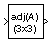• Library:
• Aerospace Blockset / Utilities / Math Operations

•## Description

The Adjoint of 3x3 Matrix block computes the adjoint matrix for the input matrix. For related equations, see Algorithms.

## Ports

### Input

expand all

Input matrix, specified as a 3-by-3 matrix, in initial acceleration units.

Data Types: `double`

### Output

expand all

Output acceleration, returned as a 3-by-3 matrix, in final acceleration units.

Data Types: `double`

## Algorithms

The input matrix has the form of

`$A=\left[\begin{array}{ccc}{A}_{11}& {A}_{12}& {A}_{13}\\ {A}_{21}& {A}_{22}& {A}_{23}\\ {A}_{31}& {A}_{32}& {A}_{33}\end{array}\right]$`

The adjoint of the matrix has the form of

`$\begin{array}{l}adj\left(A\right)=\left(\begin{array}{ccc}+|\begin{array}{cc}{A}_{22}& {A}_{23}\\ {A}_{32}& {A}_{33}\end{array}|& -|\begin{array}{cc}{A}_{12}& {A}_{13}\\ {A}_{32}& {A}_{33}\end{array}|& +|\begin{array}{cc}{A}_{12}& {A}_{13}\\ {A}_{22}& {A}_{23}\end{array}|\\ -|\begin{array}{cc}{A}_{21}& {A}_{23}\\ {A}_{31}& {A}_{33}\end{array}|& +|\begin{array}{cc}{A}_{11}& {A}_{13}\\ {A}_{31}& {A}_{33}\end{array}|& -|\begin{array}{cc}{A}_{11}& {A}_{13}\\ {A}_{21}& {A}_{23}\end{array}|\\ +|\begin{array}{cc}{A}_{21}& {A}_{22}\\ {A}_{31}& {A}_{32}\end{array}|& -|\begin{array}{cc}{A}_{11}& {A}_{12}\\ {A}_{31}& {A}_{32}\end{array}|& +|\begin{array}{cc}{A}_{11}& {A}_{12}\\ {A}_{21}& {A}_{22}\end{array}|\end{array}\right)\\ \end{array}$`

## Extended Capabilities

### C/C++ Code GenerationGenerate C and C++ code using Simulink® Coder™.